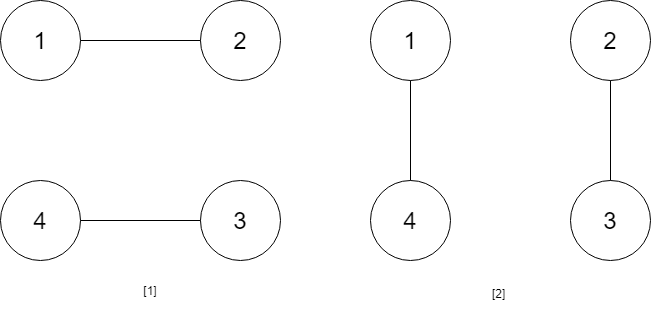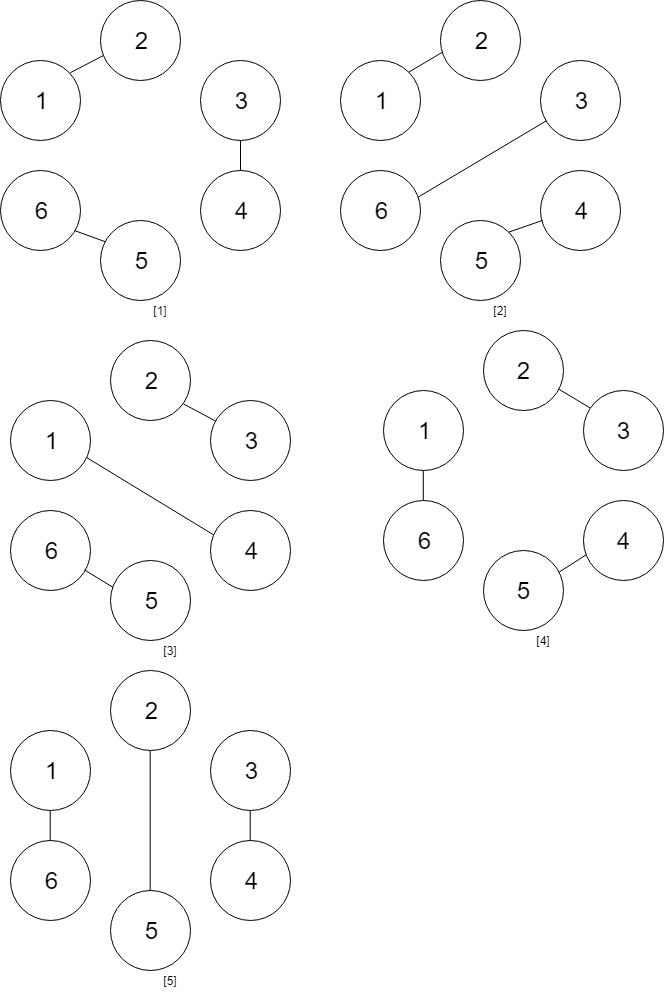Tags: "leetcode", "dynamic-programming", access_time 2-min read

# Handshakes That Dont Cross

#### Created: April 2, 2020 by [lek-tin]

##### Last updated: April 2, 2020

You are given an even number of people num_people that stand around a circle and each person shakes hands with someone else, so that there are `num_people / 2 `handshakes total.

Return the number of ways these handshakes could occur such that none of the handshakes cross.

Since this number could be very big, return the answer `mod 10^9 + 7`

### Example 1

``````Input: num_people = 2
Output: 1
``````

### Example 2``````Input: num_people = 4
Output: 2
Explanation: There are two ways to do it, the first way is [(1,2),(3,4)] and the second one is [(2,3),(4,1)].
``````

### Example 3``````Input: num_people = 6
Output: 5
``````

### Example 4

``````Input: num_people = 8
Output: 14
``````

#### Constraints

1. `2 <= num_people <= 1000`
2. `num_people % 2 == 0`

### Solution

``````class Solution:
def numberOfWays(self, num_people: int) -> int:
MOD, N = 10**9+7, num_people//2
dp =  * (N+1)
dp = 1

# dp[i] represents # of ways to shake hands when the first person shakes the ith person
for i in range(1, N+1):
# if the first person shakes hands with the jth person
# this divide people into two groups: [2:j-1] and [j+1:N]
# optimal substructure: if i shakes hands with k, f(i) = f(i) + f(j-1)*f(i-1-j)
for j in range(i):
dp[i] = (dp[i] + dp[j]*dp[i-1-j]) % MOD

return dp[N]
``````